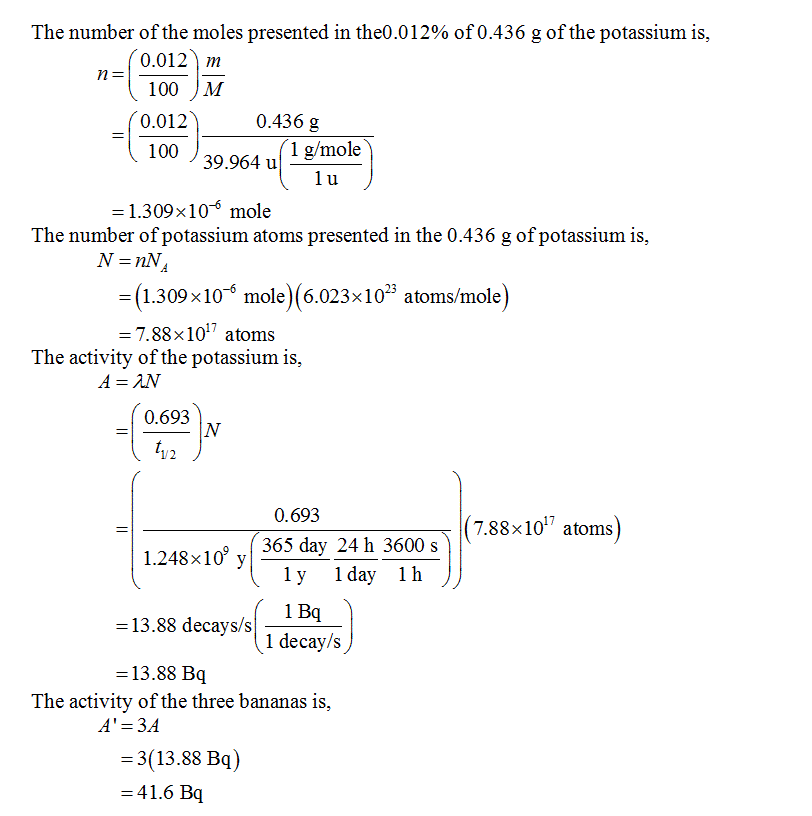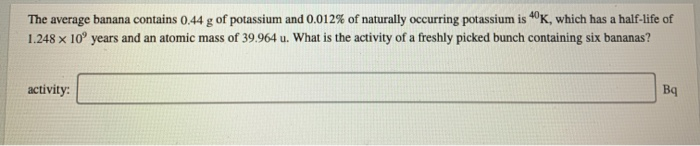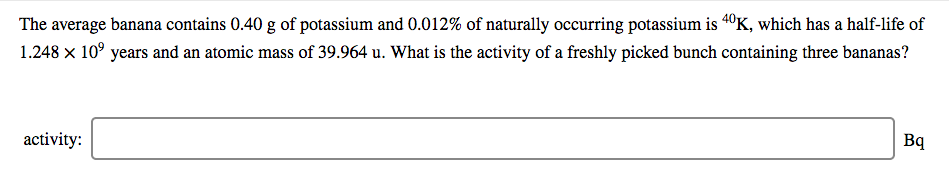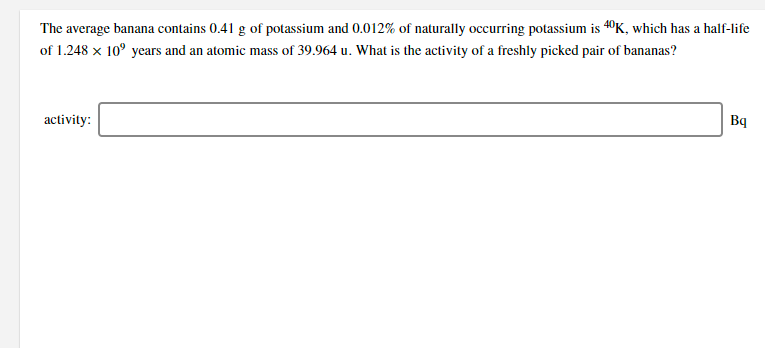Homework Help Question & Answers

# The average banana contains 0.436 g of potassium, and 0.012% of naturally occurring potassium is 40K,...

The average banana contains 0.436 g of potassium, and 0.012% of naturally occurring potassium is 40K, which has a half-life of 1.248 × 10^9 years and an atomic mass of 39.964 u. What is the activity of a freshly picked bunch containing three bananas?

The activity is the number of 40K decays that occur per unit time. Activity is commonly measured in units of Becquerel (Bq), which is defined as decays per second. Recall that the atomic mass unit u = 1.66 × 10^-24 g.

#### Homework Answers

Answer #1 ✔ Recommended AnswerAdd a comment
Know the answer?
Your Answer:

#### Post as a guest

Your Name:

What's your source?

#### Earn Coin

Coins can be redeemed for fabulous gifts.

Similar Homework Help Questions
• ### The average banana contains 0.44 g of potassium and 0.012% of naturally occurring potassium is 40K,...The average banana contains 0.44 g of potassium and 0.012% of naturally occurring potassium is 40K, which has a half-life of 1.248 x 10° years and an atomic mass of 39.964 u. What is the activity of a freshly picked bunch containing six bananas? activity: Вg

• ### The average banana contains 0.40 g of potassium and 0.012% of naturally occurring potassium is 40K,...The average banana contains 0.40 g of potassium and 0.012% of naturally occurring potassium is 40K, which has a half-life of 1.248 x 109 years and an atomic mass of 39.964 u. What is the activity of a freshly picked bunch containing three bananas? activity: Вq

• ### The average banana contains 0.41 g of potassium and 0.012% of naturally occurring potassium is 40K,...The average banana contains 0.41 g of potassium and 0.012% of naturally occurring potassium is 40K, which has a half-life of 1.248 x 10 years and an atomic mass of 39.964 u. What is the activity of a freshly picked pair of bananas? activity: Вq

Free Homework App

Scan Your Homework
to Get Instant Free Answers
Need Online Homework Help?

Get Answers For Free
Most questions answered within 3 hours.